# ICSE Class 10 Physics Question Paper Solution 2017

Solving ICSE Class 10 Physics Question Paper 2017 helps students in understanding the difficulty level of the exam and the way questions are asked in the ICSE 10th exam. Also, they get to know the paper pattern thoroughly. In case they are stuck somewhere while solving the paper, they can refer to the ICSE Class 10 Physics Question Paper Solution 2017. The solution pdf contains the answers to all questions explained in detail, along with the marking scheme. By referring to the solutions, students will also understand how marks are allocated for each step.

The ICSE Class 10 Physics 2017 exam was conducted on 27th March 2017. The exam started at 11 am and students were allotted 2 hours of time duration to finish the paper. Students can download the ICSE Class 10 Physics Question Paper Solution 2017 PDF from the link below.

Students can have a look at the ICSE Class 10 Physics Question Paper Solution 2017 below: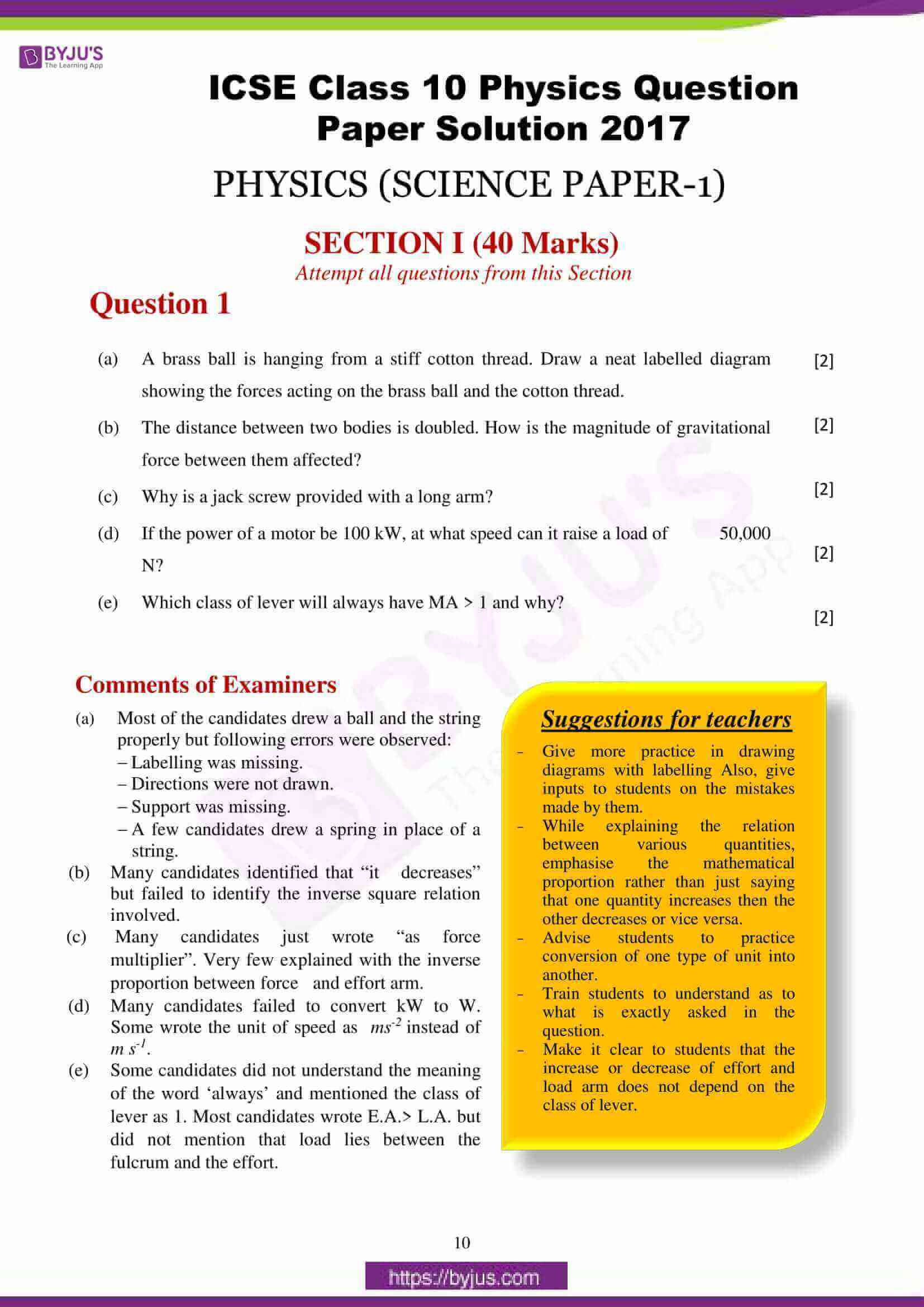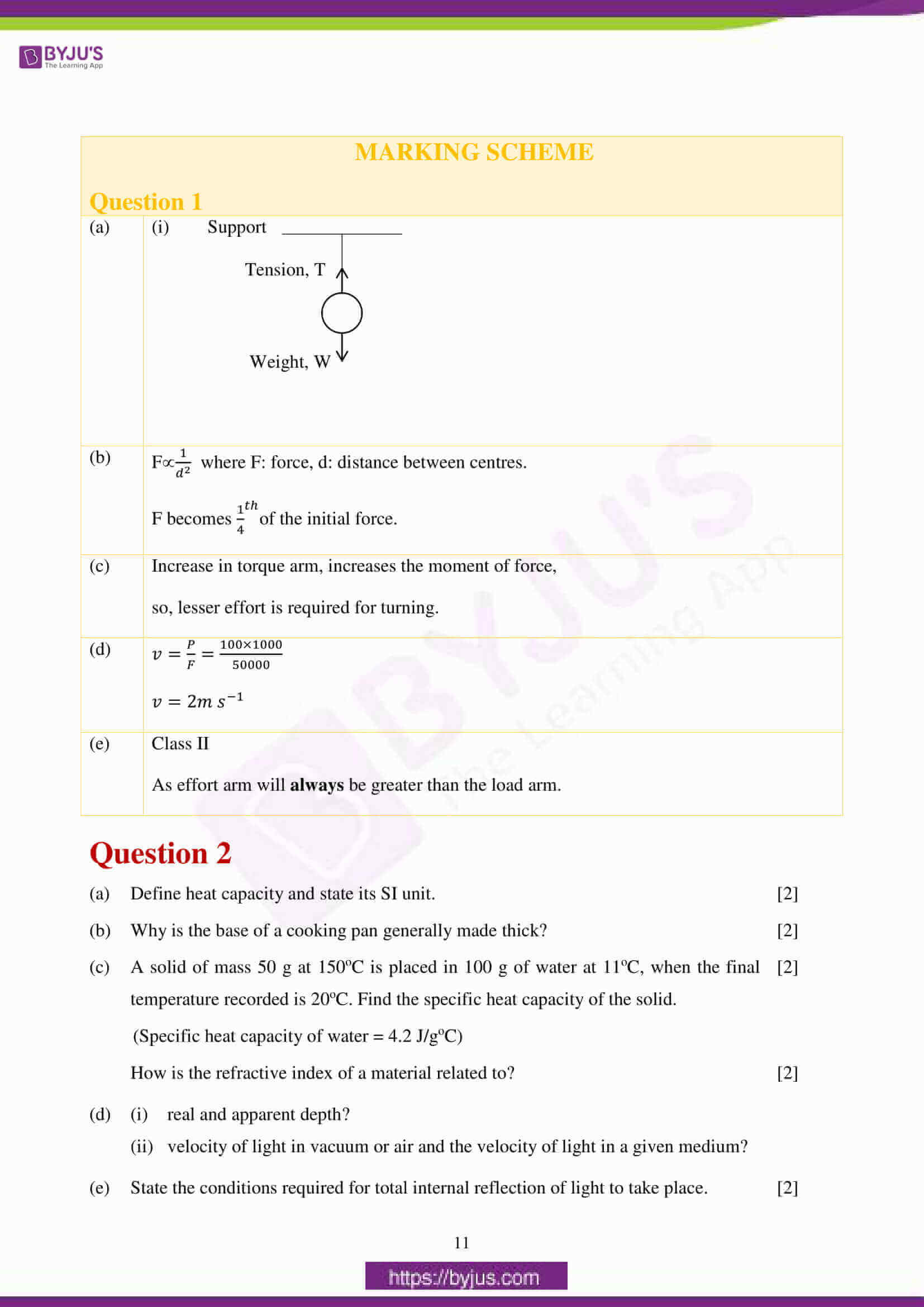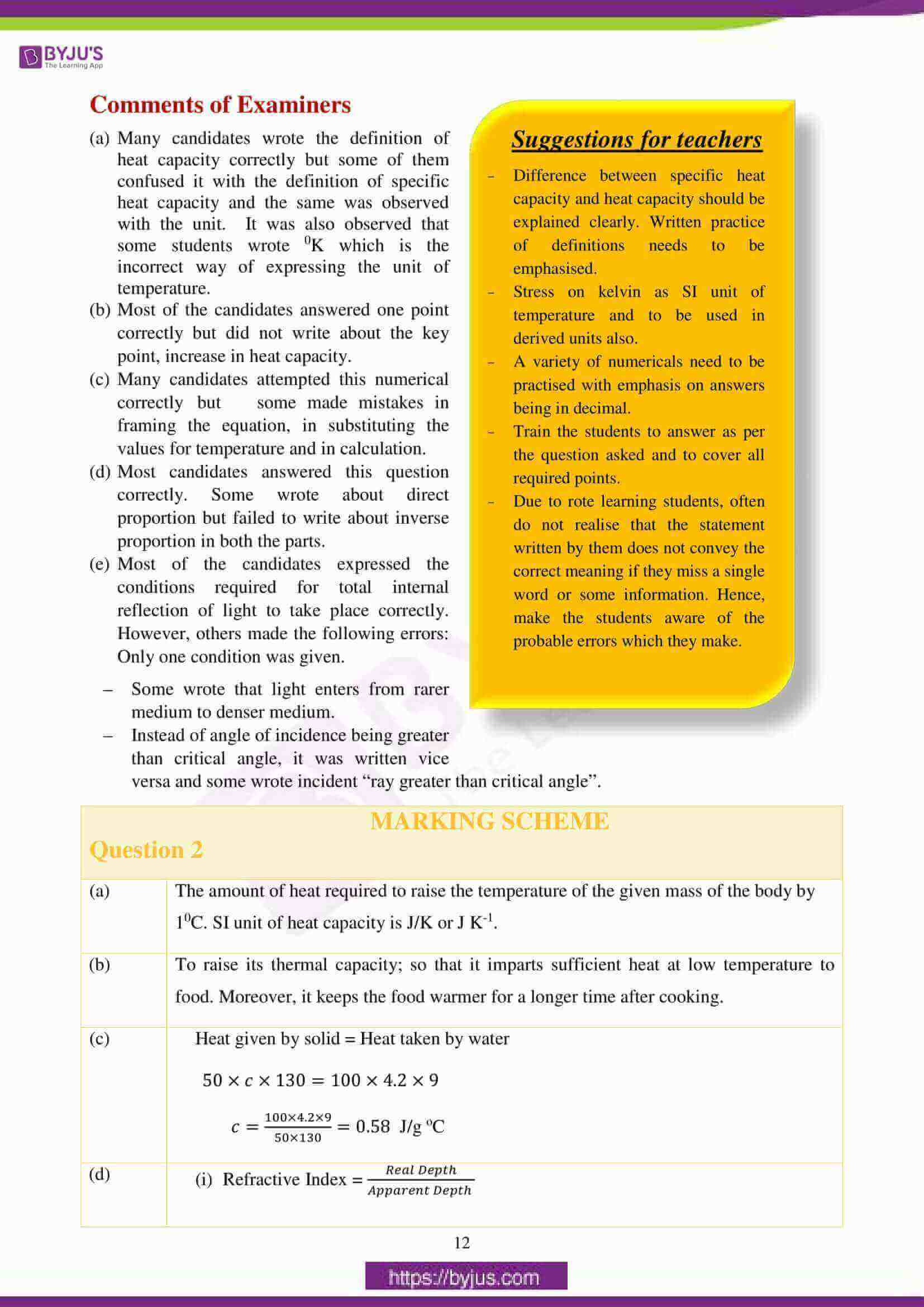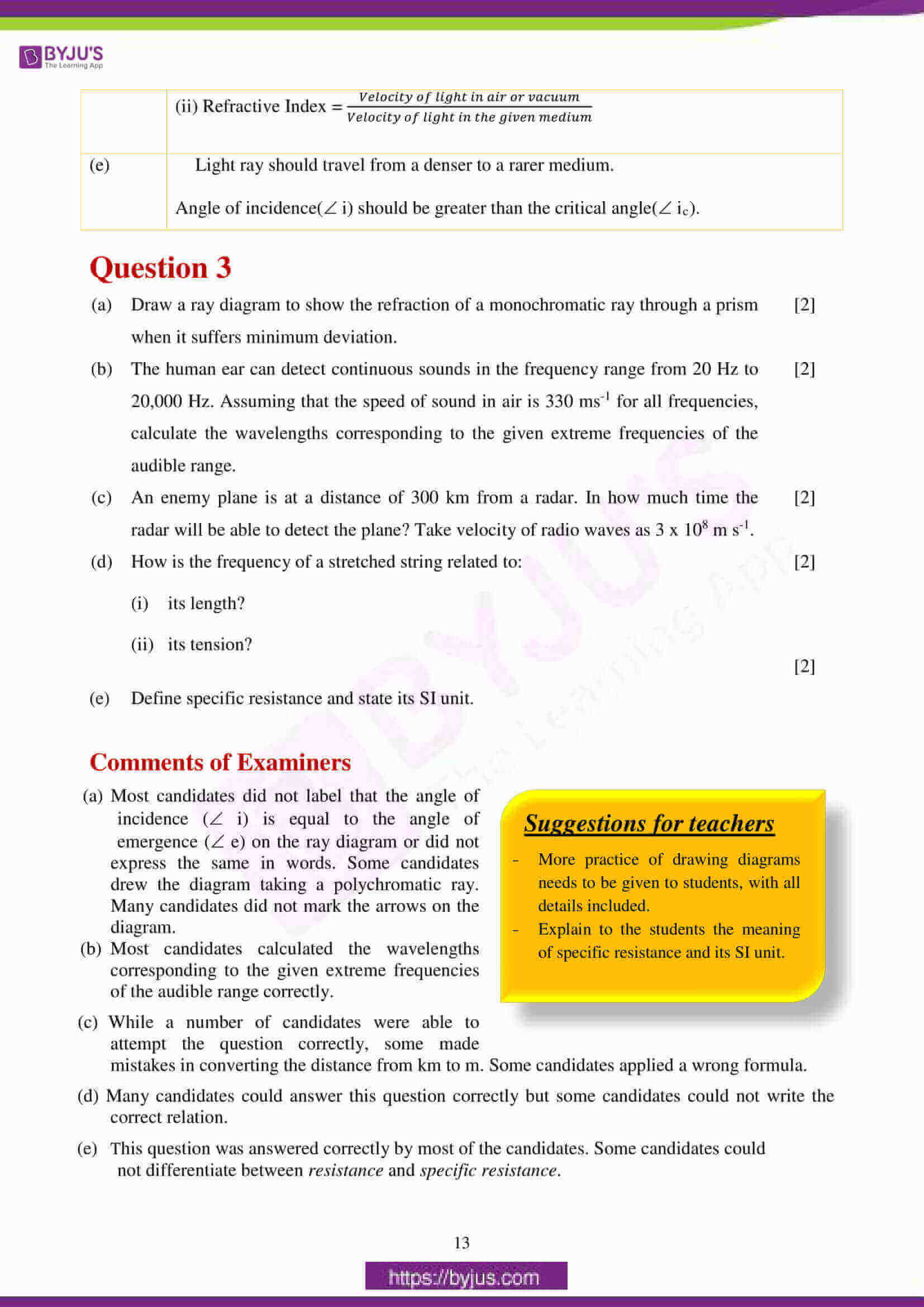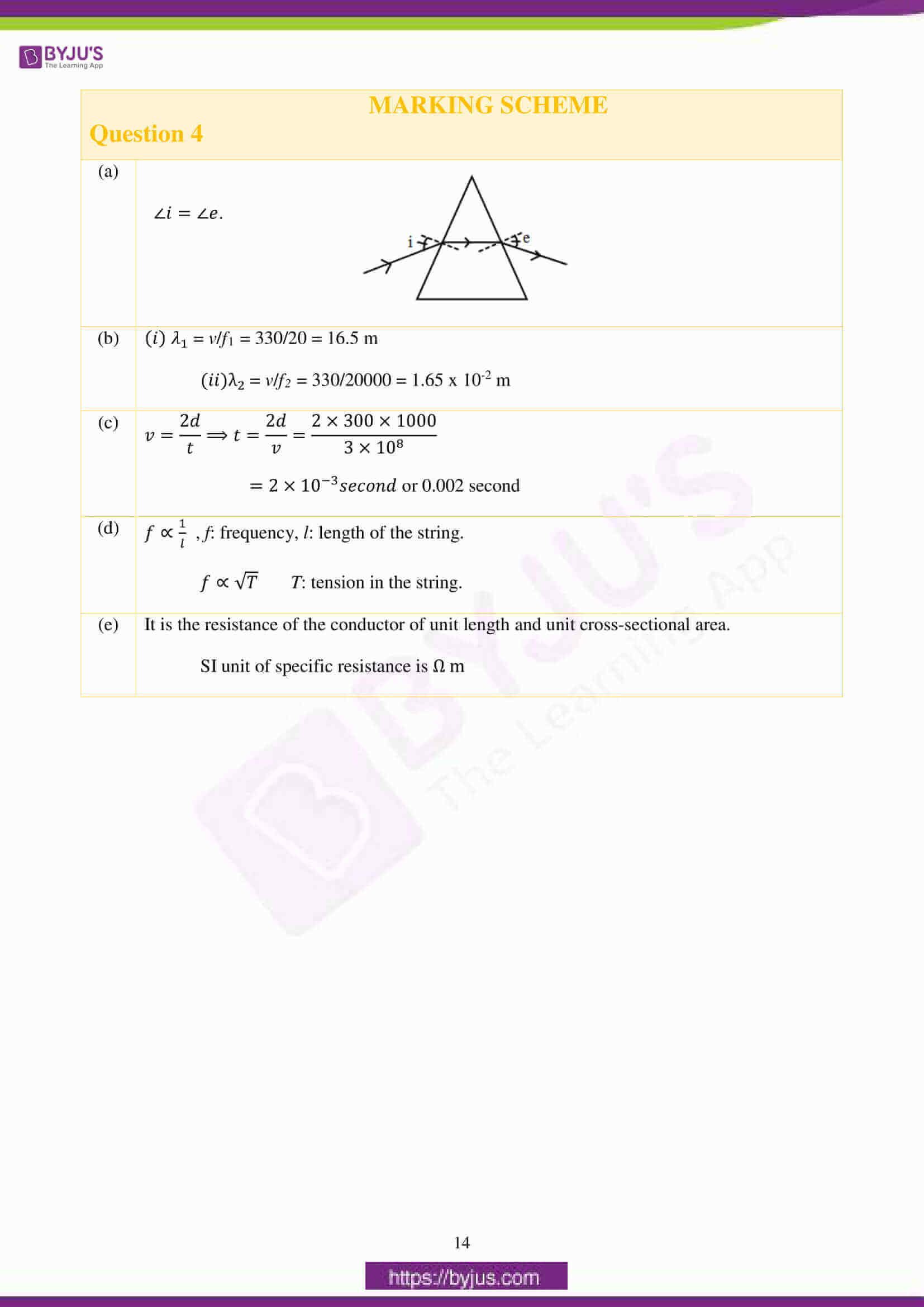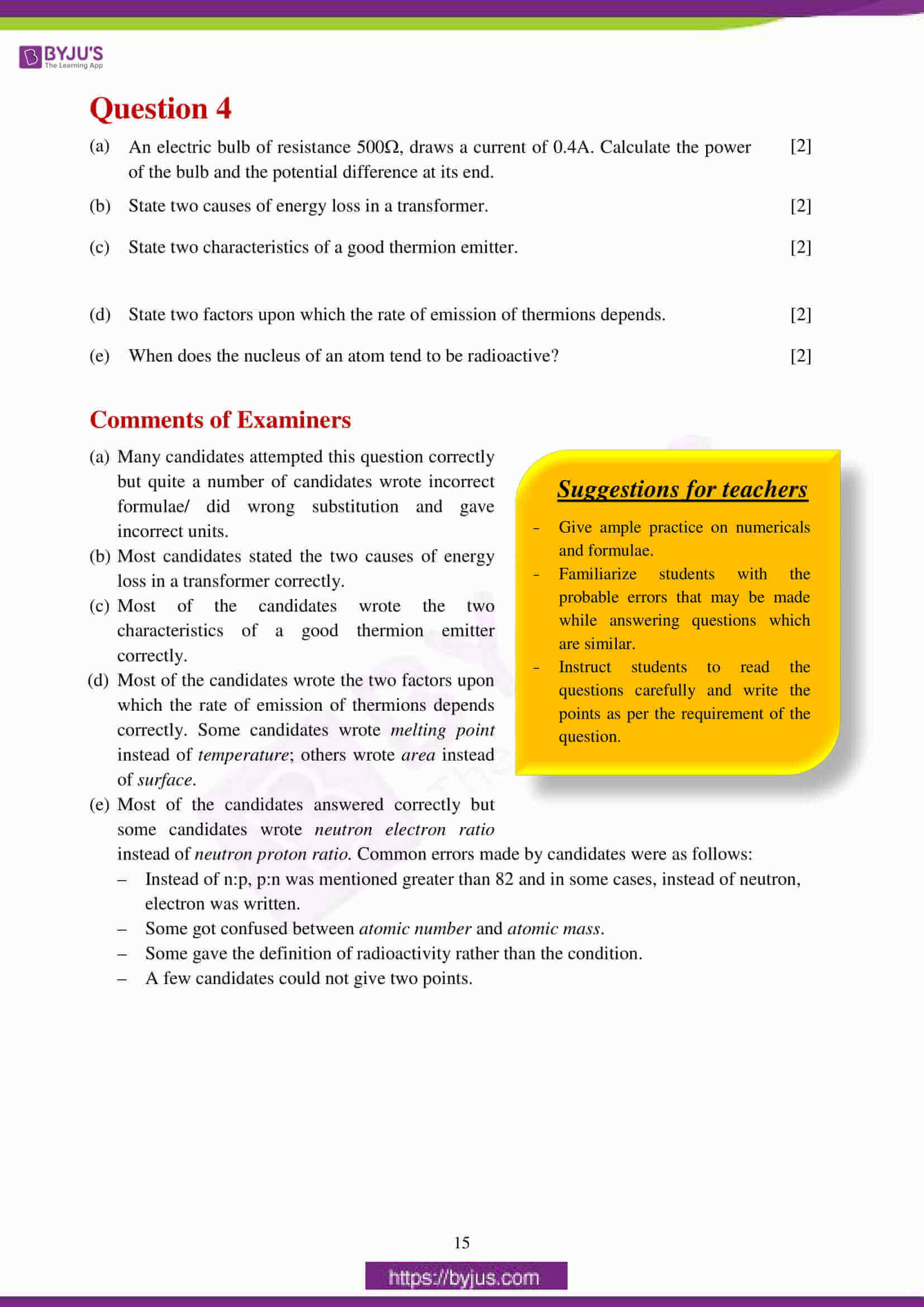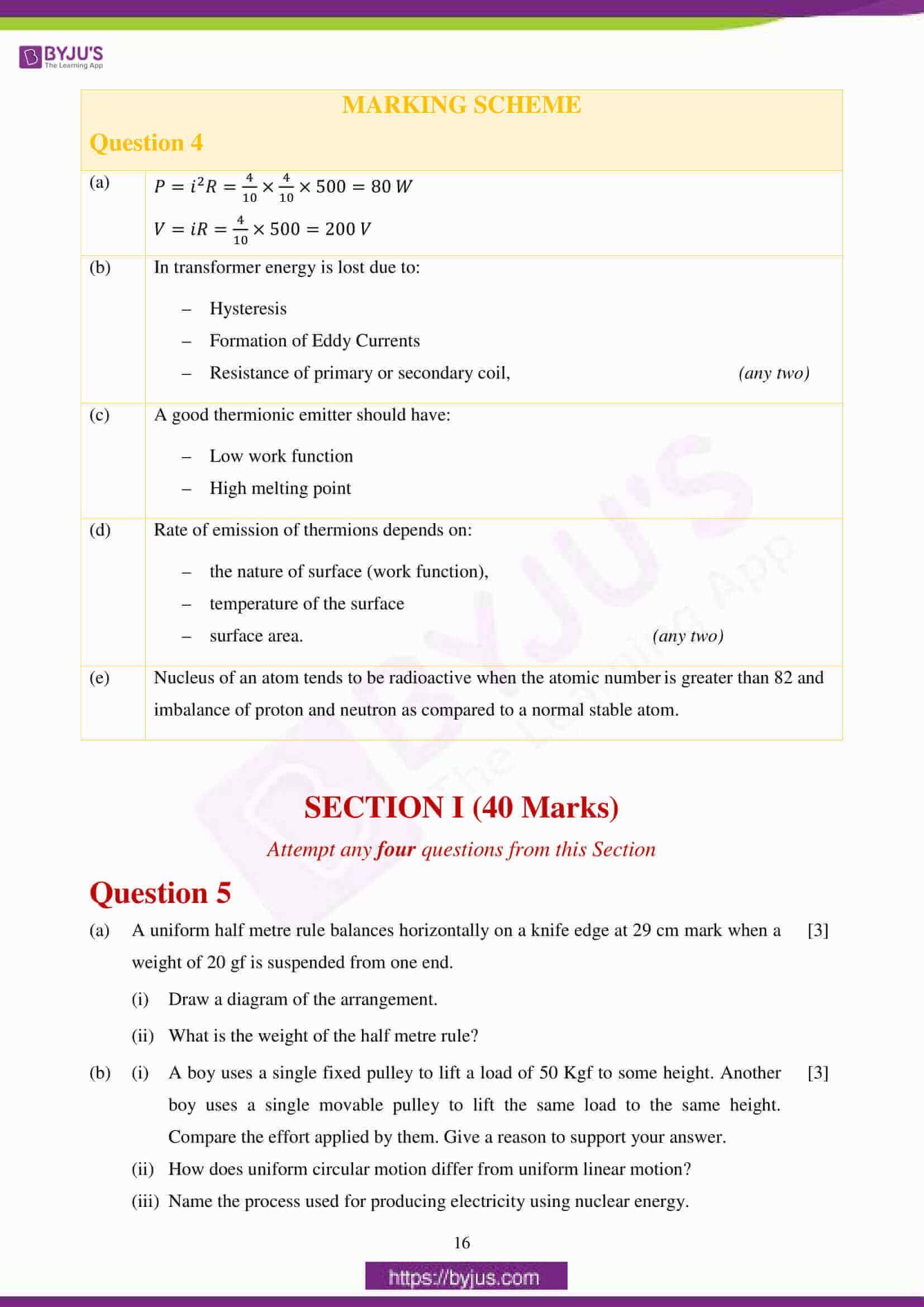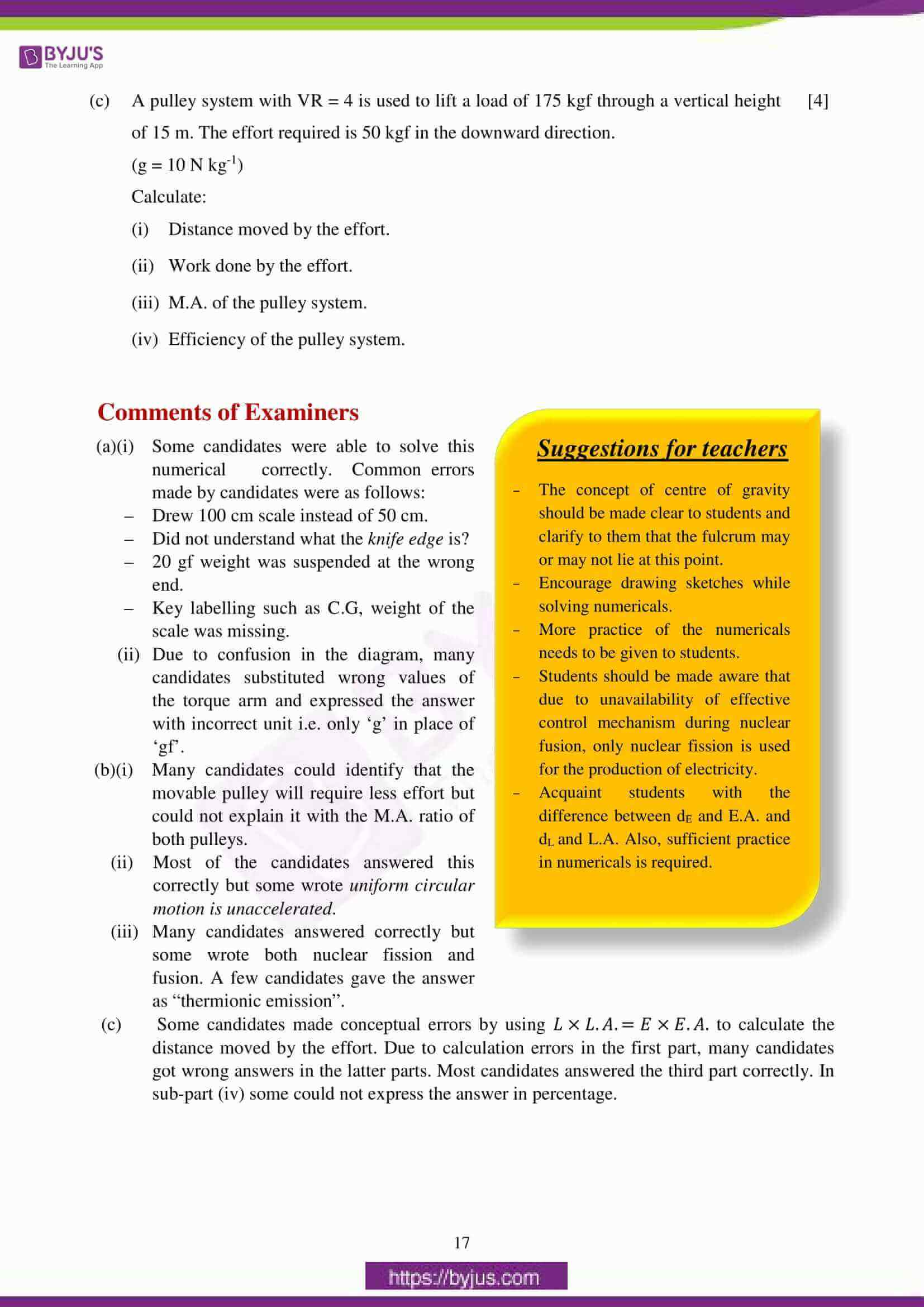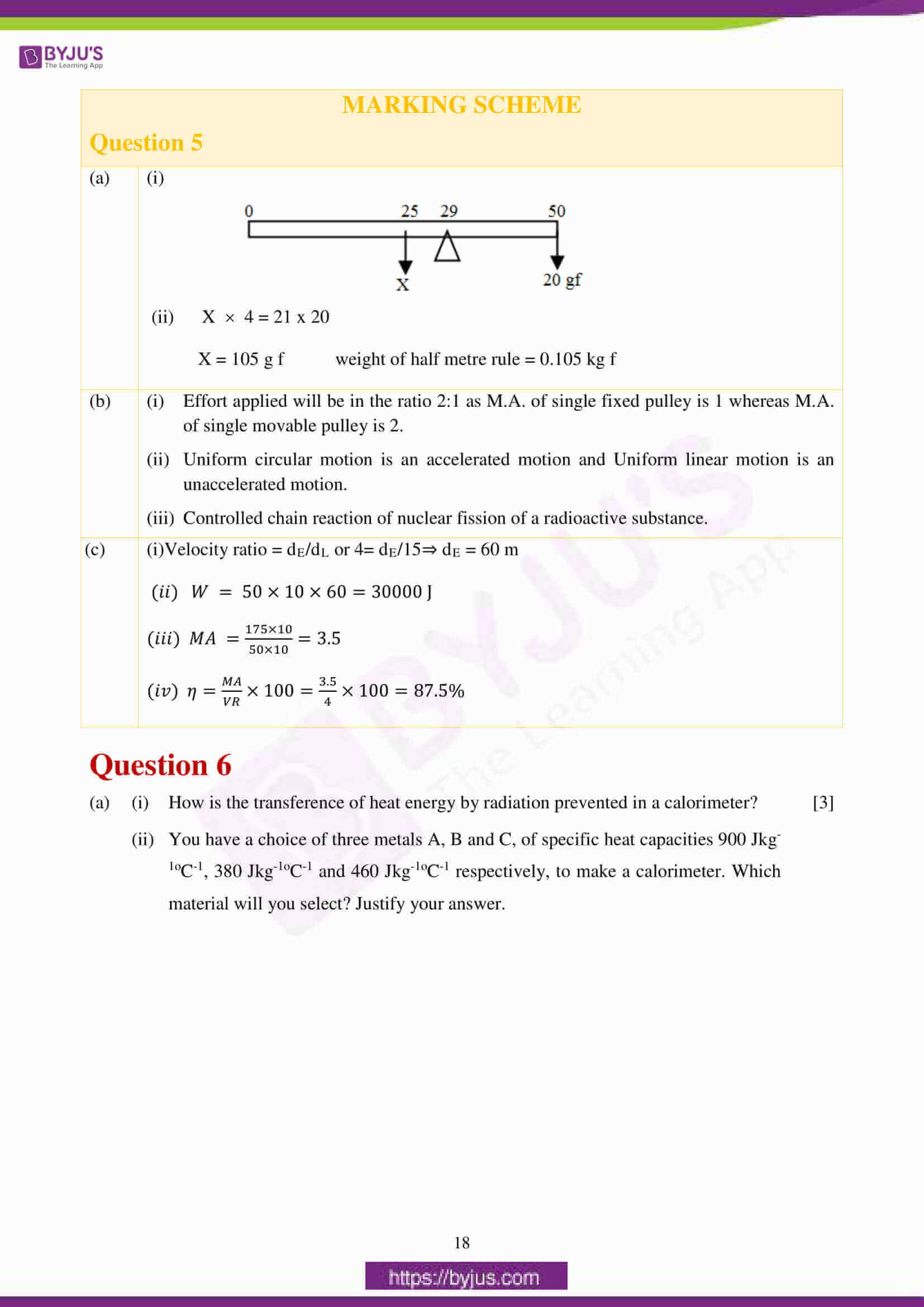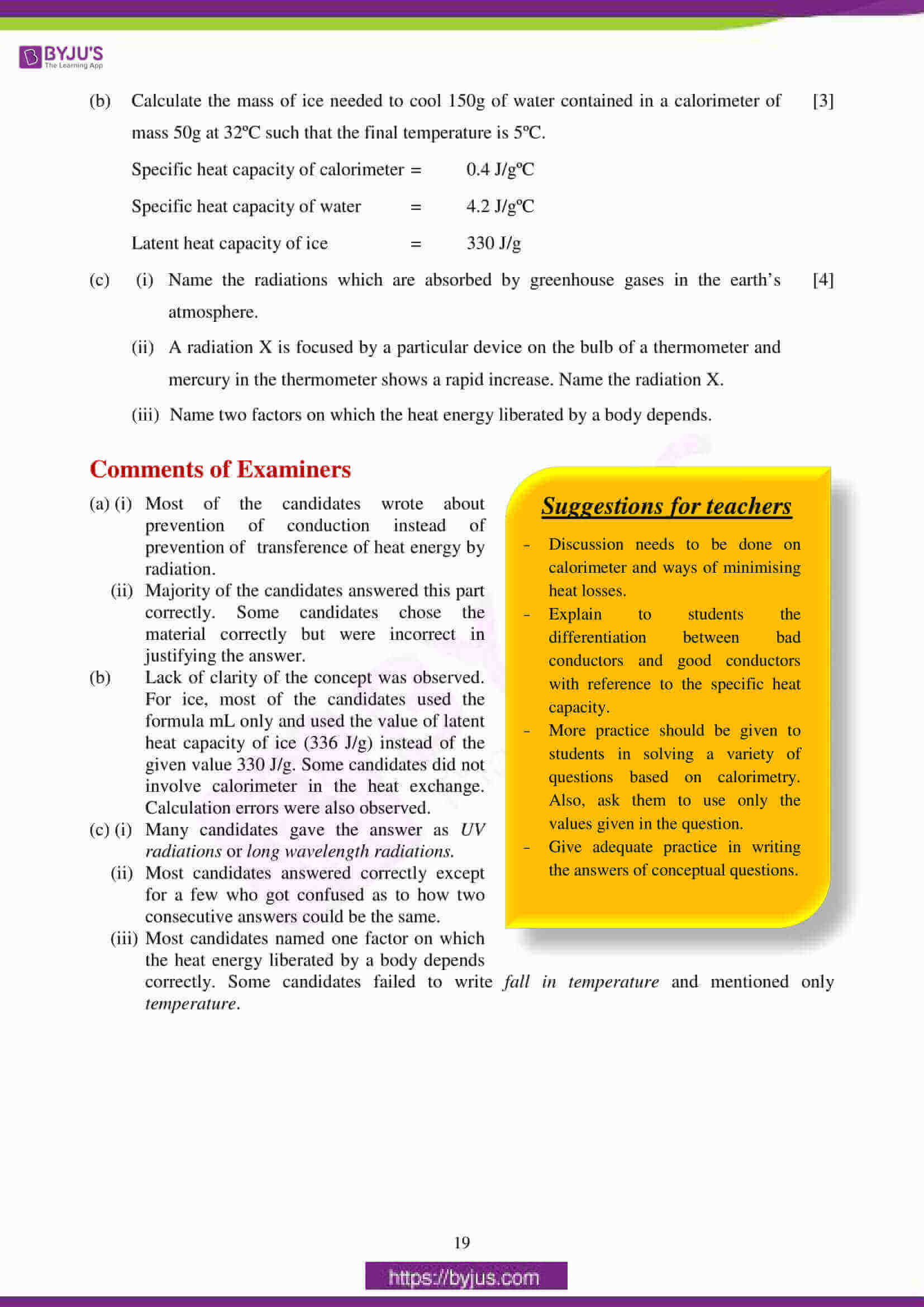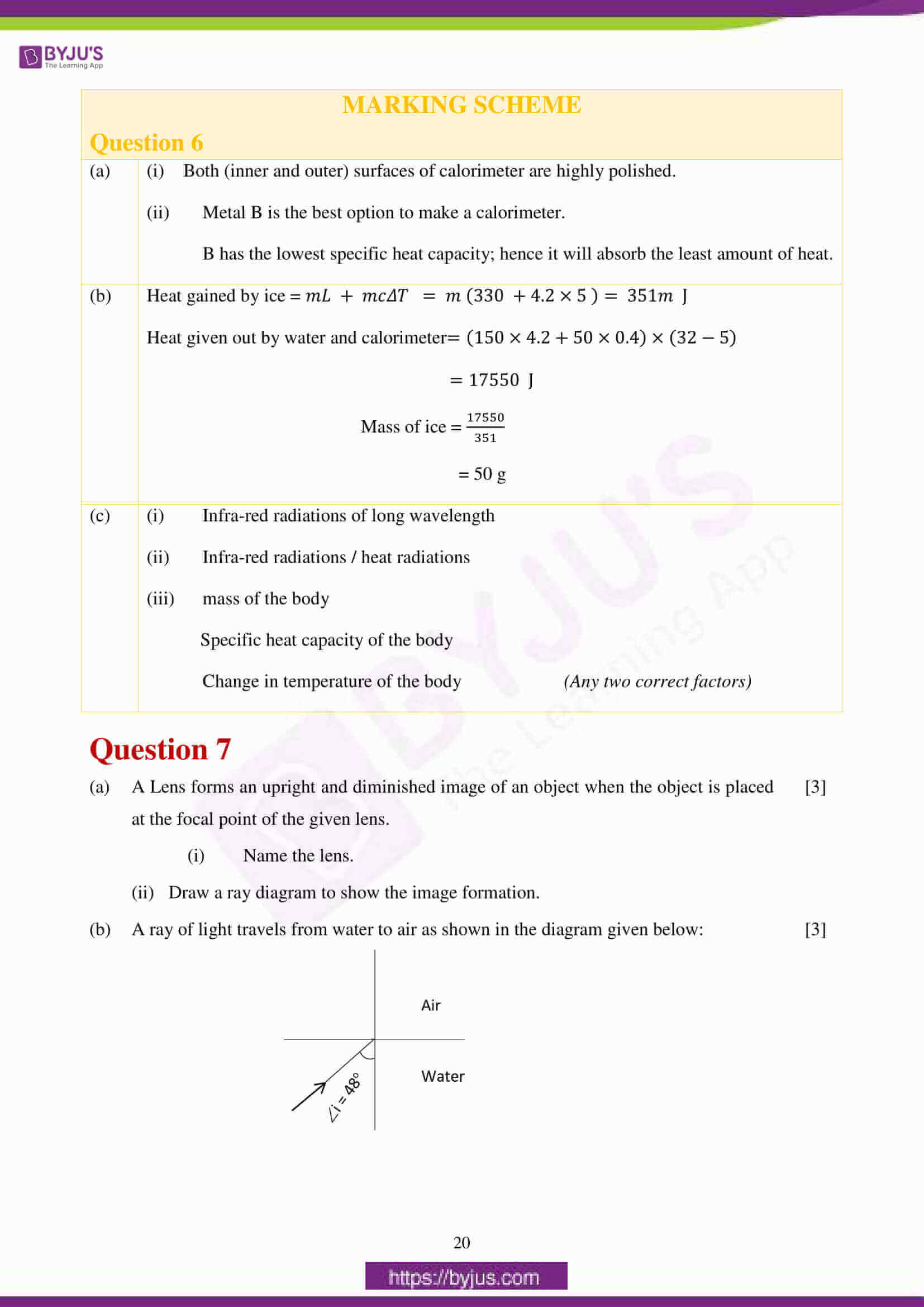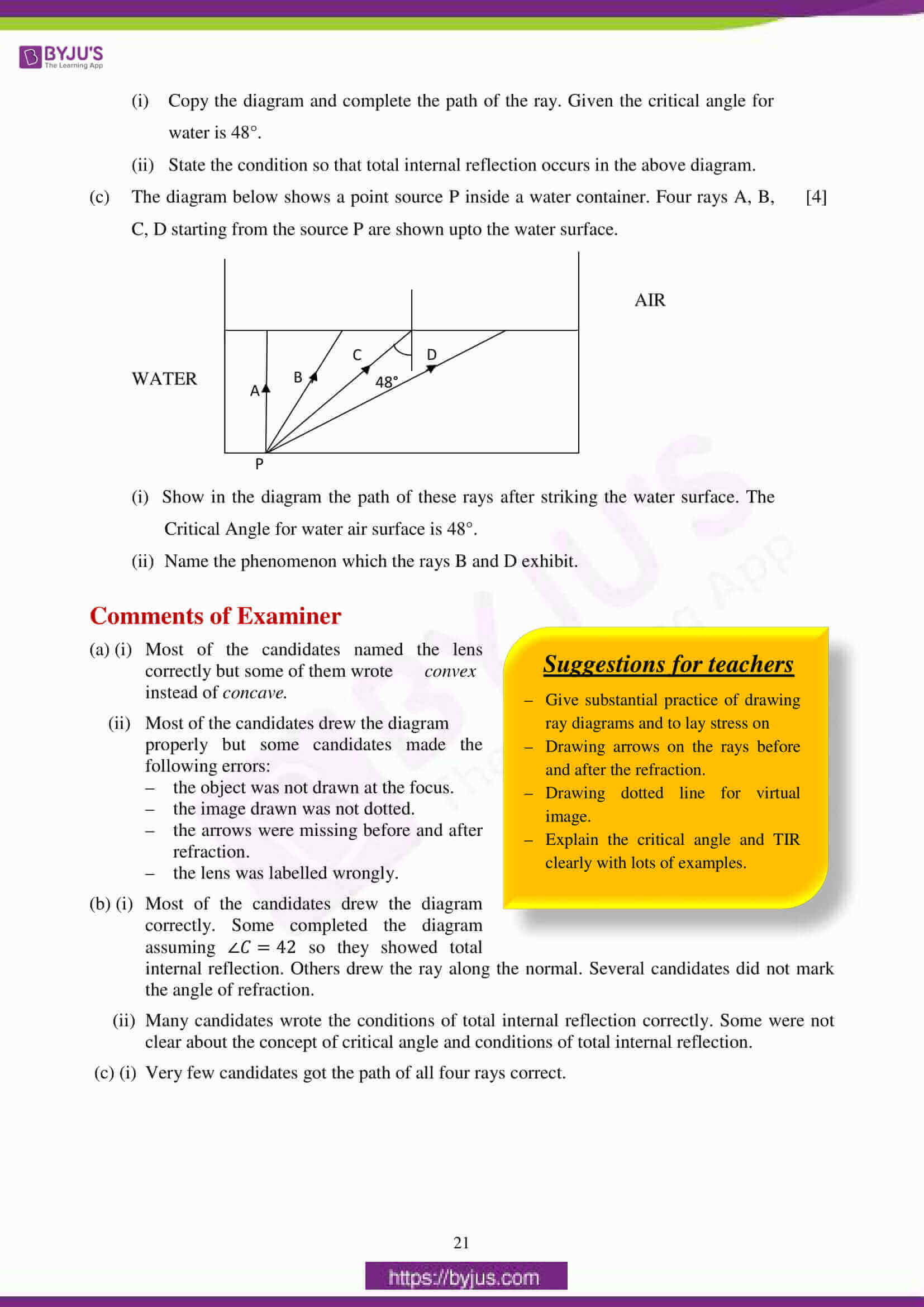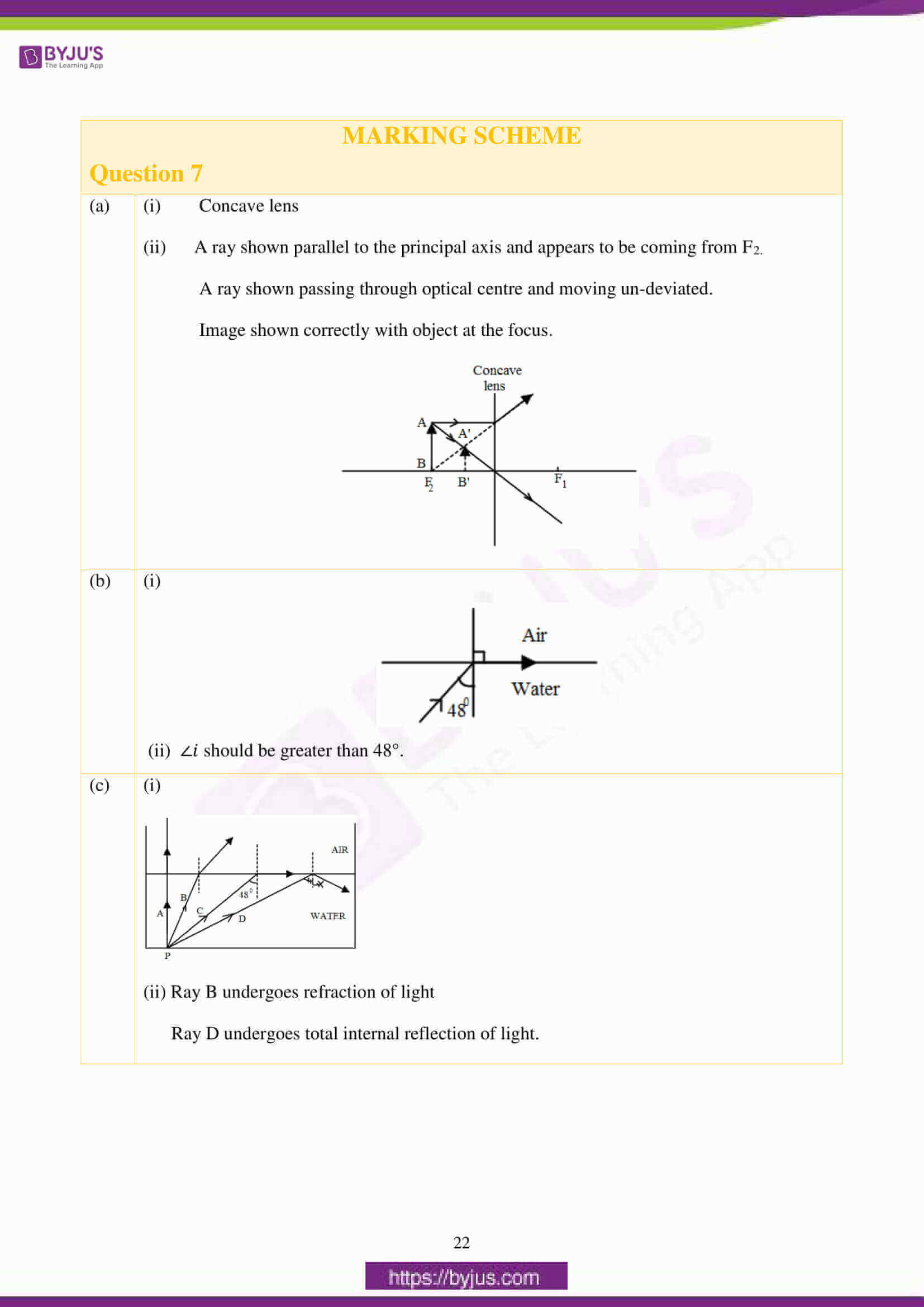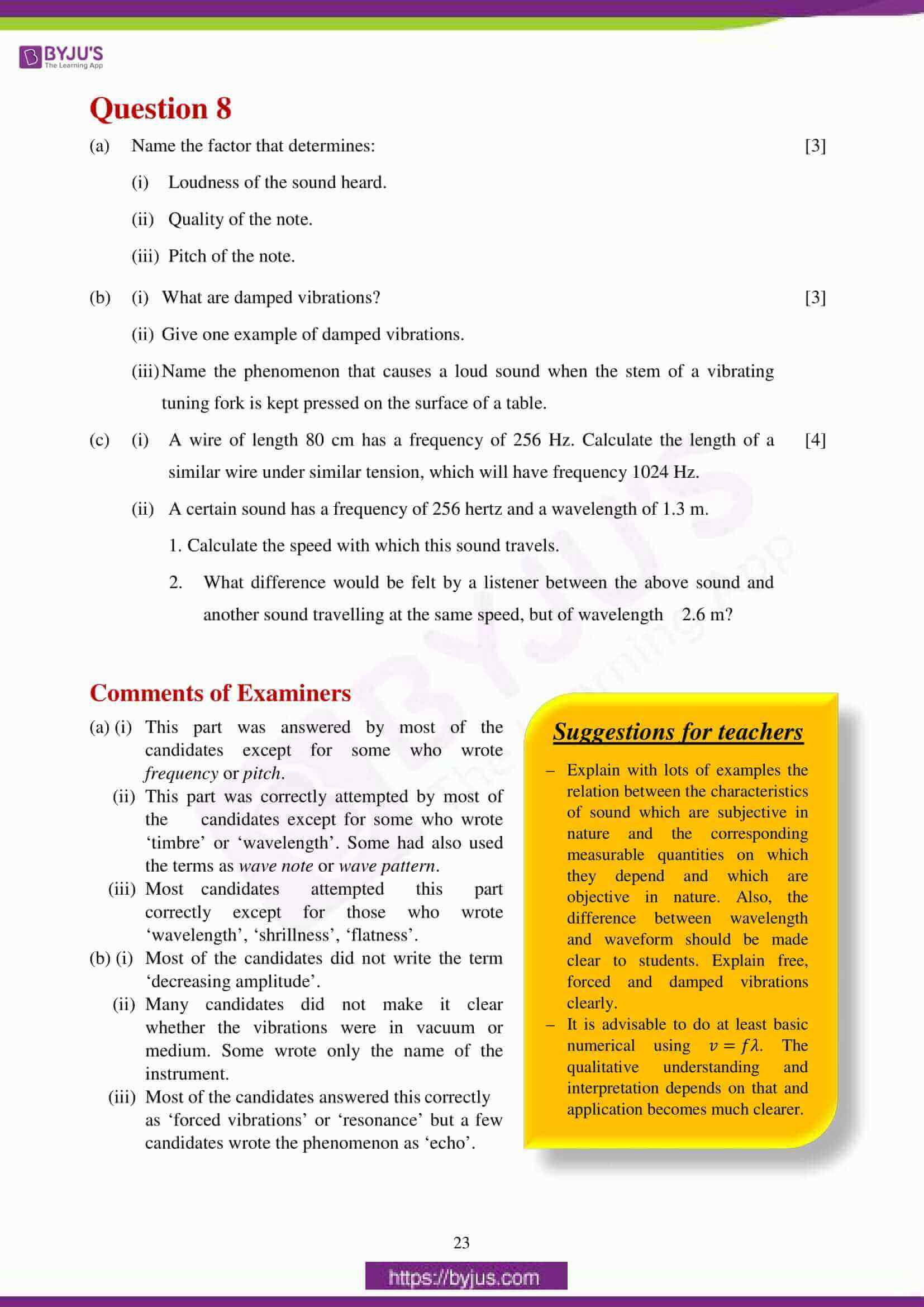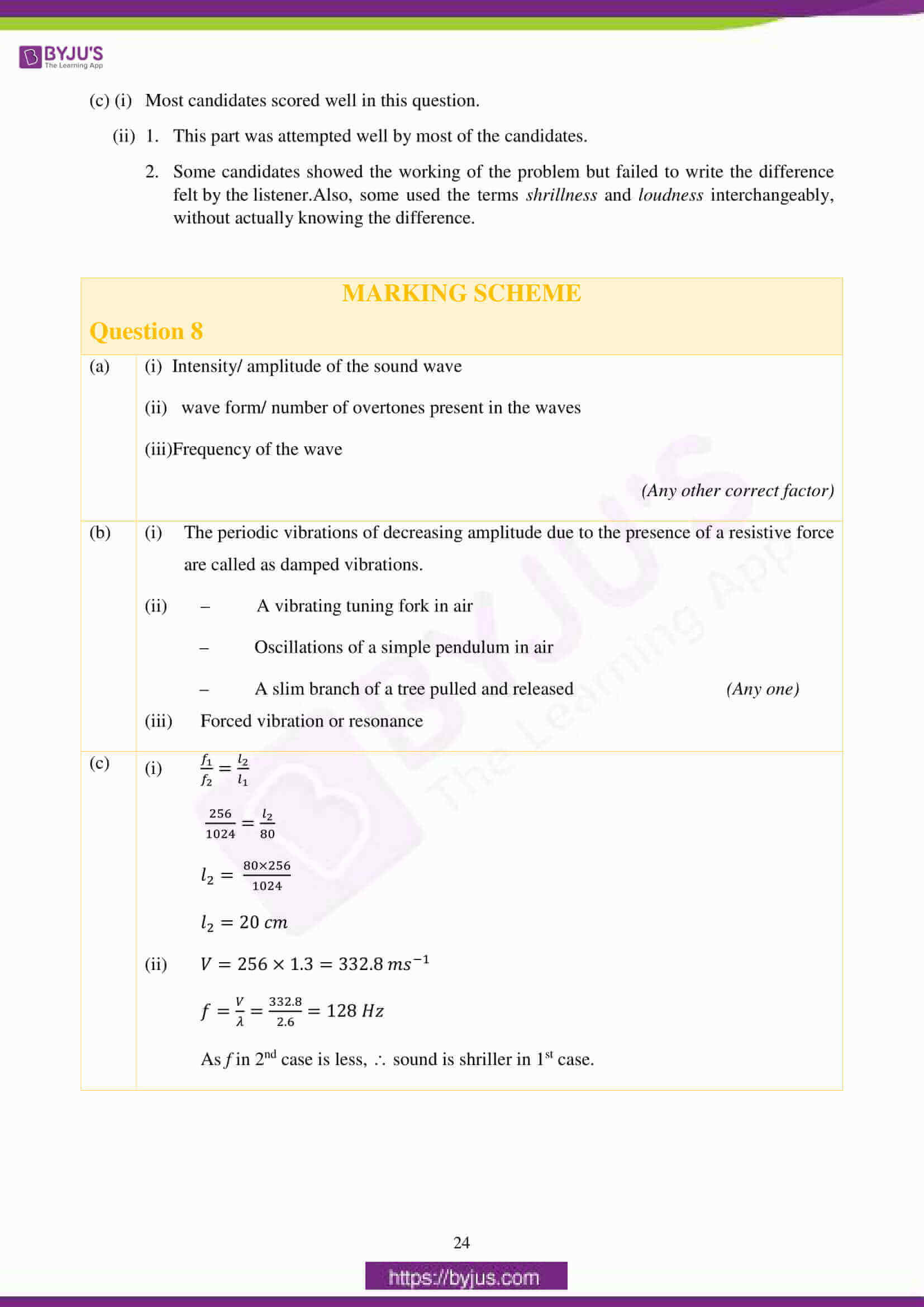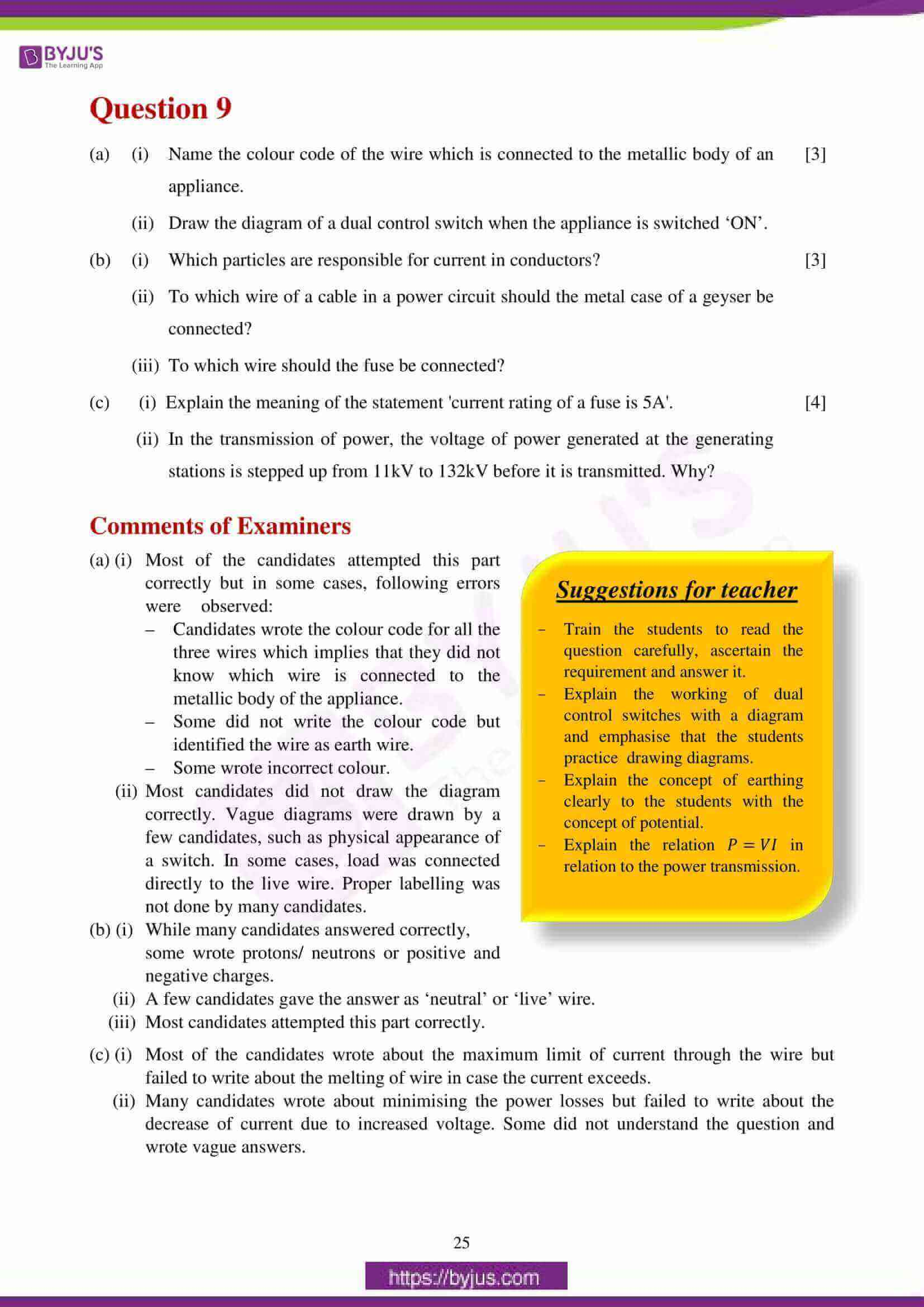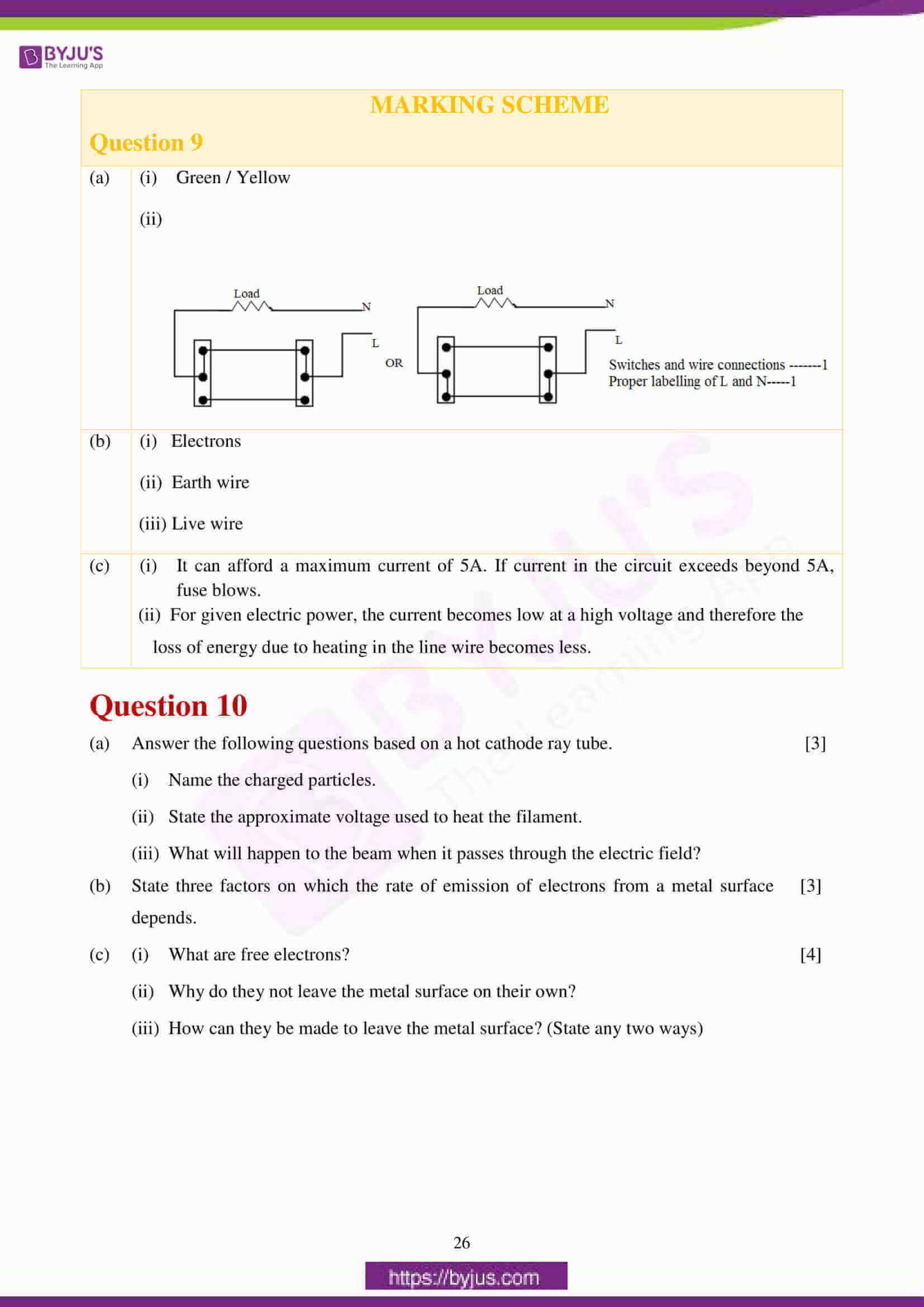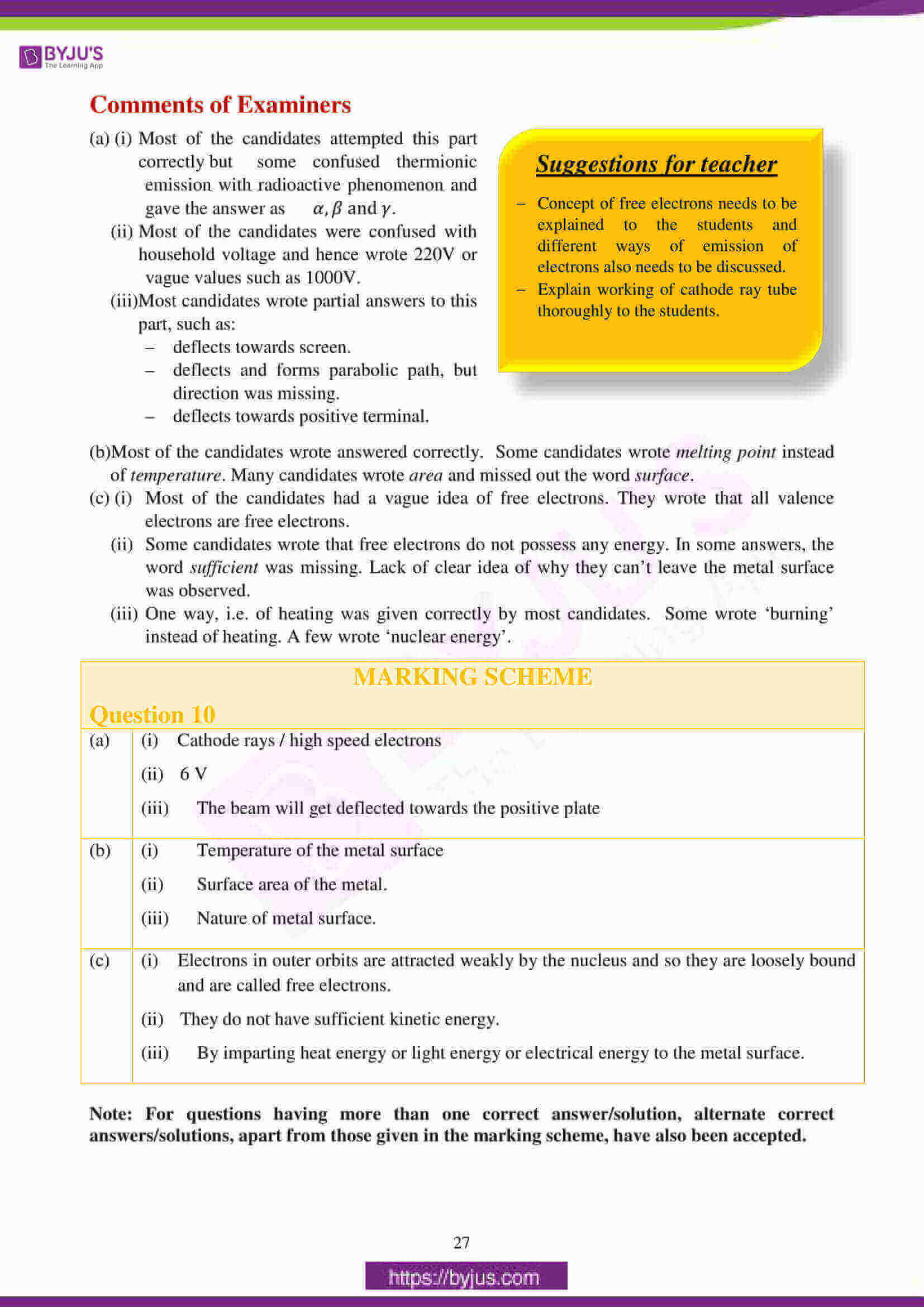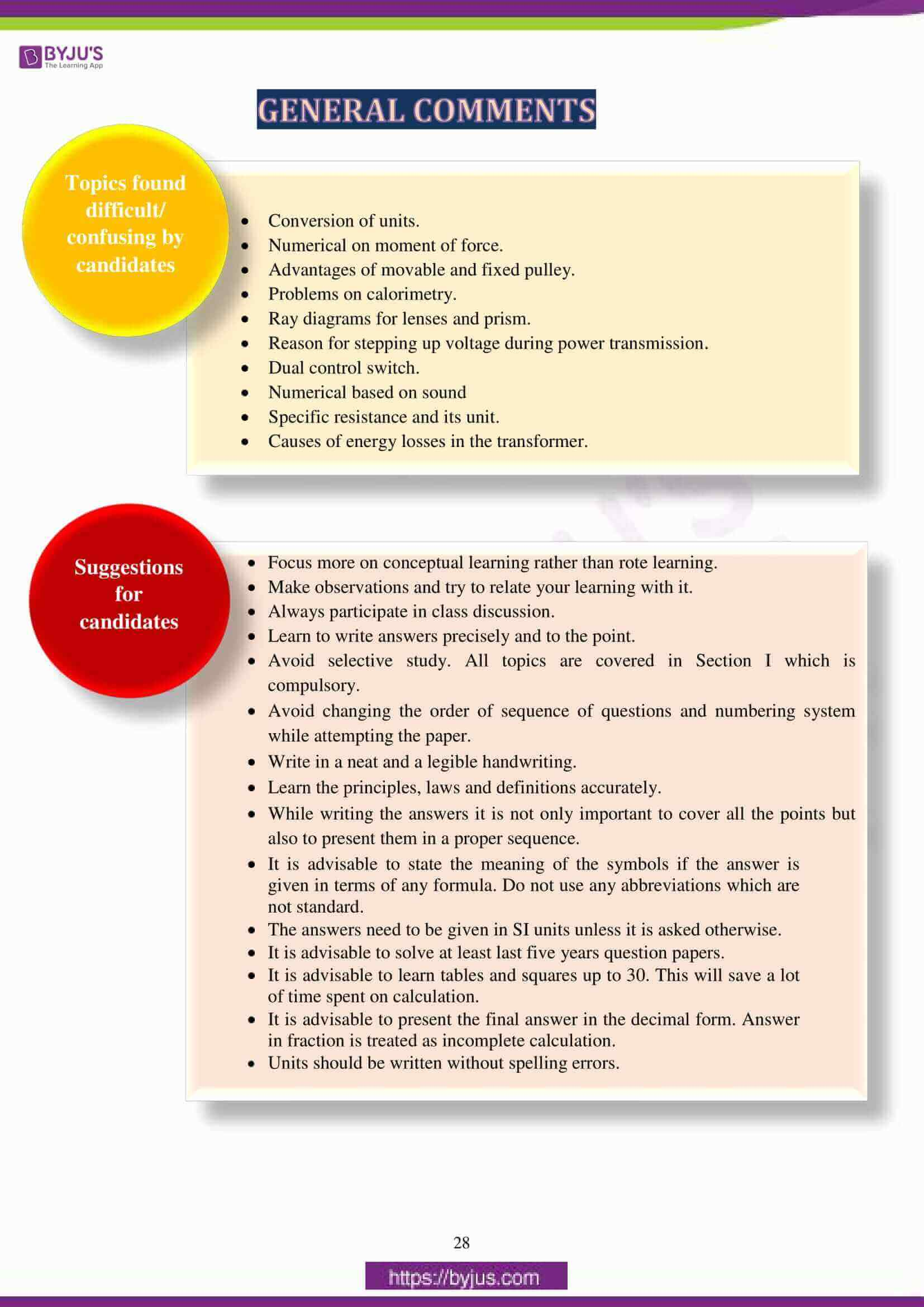## ICSE Class 10 Physics (Science Paper 1) Question Paper 2017 With Solution

### Question 1:

(a) A brass ball is hanging from a stiff cotton thread. Draw a neat labelled diagram showing the forces acting on the brass ball and the cotton thread.

(b) The distance between two bodies is doubled. How is the magnitude of gravitational force between them affected?

(c) Why is a jack screw provided with a long arm?

(d) If the power of a motor be 100 kW, at what speed can it raise a load of 50,000 N?

(e) Which class of lever will always have MA > 1 and why?

(a) (i)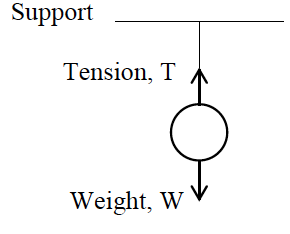(b) F∝ 1/𝑑2 where F: force, d: distance between centres.

F becomes 1/4th of the initial force of the initial force.

(c) Increase in torque arm, increases the moment of force, so, lesser effort is required for turning.

(d) 𝑣 = 𝑃/𝐹 = 100×1000/50000

𝑣= 2𝑚𝑠−1

(e) Class II

As effort arm will always be greater than the load arm.

### Question 2:

(a) Define heat capacity and state its SI unit.

(b) Why is the base of a cooking pan generally made thick?

(c) A solid of mass 50 g at 150oC is placed in 100 g of water at 11oC, when the final temperature recorded is 20oC. Find the specific heat capacity of the solid. (Specific heat capacity of water = 4.2 J/goC)

(d) How is the refractive index of a material related to?

(i) real and apparent depth?

(ii) velocity of light in vacuum or air and the velocity of light in a given medium?

(e) State the conditions required for total internal reflection of light to take place.

(a) The amount of heat required to raise the temperature of the given mass of the body by 10C. SI unit of heat capacity is J/K or J K-1.

(b) To raise its thermal capacity; so that it imparts sufficient heat at low temperature to food. Moreover, it keeps the food warmer for a longer time after cooking.

(c) Heat given by solid = Heat taken by water

50 × c × 130 = 100 × 4.2 × 9

c = (100 × 4.2 × 9)/(50 × c × 130) = 0.58 J/g oC

(d) (i) Refractive Index = Real depth / Apparent Depth

(ii) Refractive Index = Velocity of light in air or vacuum / Velocity of light in the given medium

(e) Light ray should travel from a denser to a rarer medium.

Angle of incidence(∠ i) should be greater than the critical angle(∠ ic).

### Question 3:

(a) Draw a ray diagram to show the refraction of a monochromatic ray through a prism when it suffers minimum deviation.

(b) The human ear can detect continuous sounds in the frequency range from 20 Hz to 20,000 Hz. Assuming that the speed of sound in air is 330 ms-1 for all frequencies, calculate the wavelengths corresponding to the given extreme frequencies of the audible range.

(c) An enemy plane is at a distance of 300 km from a radar. In how much time the radar will be able to detect the plane? Take velocity of radio waves as 3 x 108 m s-1.

(d) How is the frequency of a stretched string related to:

(i) its length?

(ii) its tension?

(e) Define specific resistance and state its SI unit.

(a)

∠𝑖 = ∠𝑒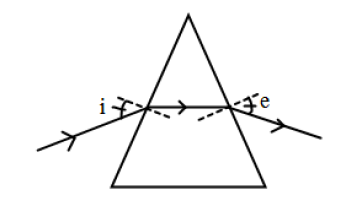(b) (𝑖) 𝜆1 = v/f1 = 330/20 = 16.5 m

(𝑖𝑖) λ2 = v/f2 = 330/20000 = 1.65 x 10-2 m

(c) 𝑣 = 2𝑑/𝑡

⟹ 𝑡 = 2𝑑/𝑣 = (2 × 300 × 1000) / (3 × 108)

= 2 × 10−3 or 0.002 second

(d) 𝑓∝ 1/𝑙 , f: frequency, l: length of the string.

𝑓∝ √𝑇

T: tension in the string.

(e) It is the resistance of the conductor of unit length and unit cross-sectional area. SI unit of specific resistance is Ω m

### Question 4:

(a) An electric bulb of resistance 500Ω, draws a current of 0.4A. Calculate the power of the bulb and the potential difference at its end.

(b) State two causes of energy loss in a transformer.

(c) State two characteristics of a good thermion emitter.

(d) State two factors upon which the rate of emission of thermions depends.

(e) When does the nucleus of an atom tend to be radioactive?

(a)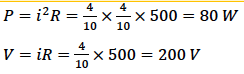(b) In transformer energy is lost due to:

– Hysteresis

– Formation of Eddy Currents

– Resistance of primary or secondary coil, (any two)

(c) A good thermionic emitter should have:

– Low work function

– High melting point

(d) Rate of emission of thermions depends on:

– the nature of surface (work function),

– temperature of the surface

– surface area. (any two)

(e) Nucleus of an atom tends to be radioactive when the atomic number is greater than 82 and imbalance of proton and neutron as compared to a normal stable atom.

### Question 5:

(a) A uniform half metre rule balances horizontally on a knife edge at 29 cm mark when a weight of 20 gf is suspended from one end.

(i) Draw a diagram of the arrangement.

(ii) What is the weight of the half metre rule?

(b) (i) A boy uses a single fixed pulley to lift a load of 50 Kgf to some height. Another boy uses a single movable pulley to lift the same load to the same height. Compare the effort applied by them. Give a reason to support your answer.

(ii) How does uniform circular motion differ from uniform linear motion?

(iii) Name the process used for producing electricity using nuclear energy.

(c) A pulley system with VR = 4 is used to lift a load of 175 kgf through a vertical height of 15 m. The effort required is 50 kgf in the downward direction. (g = 10 N kg-1)

Calculate:

(i) Distance moved by the effort.

(ii) Work done by the effort.

(iii) M.A. of the pulley system.

(iv) Efficiency of the pulley system.

(a) (i)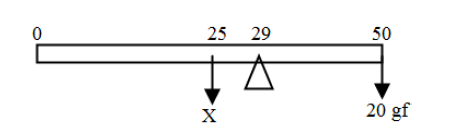(ii) X × 4 = 21 x 20

X = 105 g f

weight of half metre rule = 0.105 kg f

(b) (i) Effort applied will be in the ratio 2:1 as M.A. of single fixed pulley is 1 whereas M.A. of single movable pulley is 2.

(ii) Uniform circular motion is an accelerated motion and Uniform linear motion is an unaccelerated motion.

(iii) Controlled chain reaction of nuclear fission of a radioactive substance.

(c) (i) Velocity ratio = dE/dL or 4 = dE/15 ⇒ dE = 60 m

(𝑖𝑖) 𝑊 = 50 × 10 × 60 = 30000 J

(𝑖𝑖𝑖) 𝑀A = (175 × 10) / (50 × 10) = 3.5

(𝑖v) 𝜂 = 𝑀A / VR × 100 = 3.5 / 4 × 100 = 87.5%

### Question 6:

(a) (i) How is the transference of heat energy by radiation prevented in a calorimeter?

(ii) You have a choice of three metals A, B and C, of specific heat capacities 900 Jkg-1oC-1, 380 Jkg-1oC-1 and 460 Jkg-1oC-1 respectively, to make a calorimeter. Which material will you select? Justify your answer.

(b) Calculate the mass of ice needed to cool 150g of water contained in a calorimeter of mass 50g at 32ºC such that the final temperature is 5ºC.

Specific heat capacity of calorimeter = 0.4 J/gºC

Specific heat capacity of water = 4.2 J/gºC

Latent heat capacity of ice = 330 J/g

(c) (i) Name the radiations which are absorbed by greenhouse gases in the earth’s atmosphere.

(ii) A radiation X is focused by a particular device on the bulb of a thermometer and mercury in the thermometer shows a rapid increase. Name the radiation X.

(iii) Name two factors on which the heat energy liberated by a body depends.

(a) (i) Both (inner and outer) surfaces of calorimeter are highly polished.

(ii) Metal B is the best option to make a calorimeter.

B has the lowest specific heat capacity; hence it will absorb the least amount of heat.

(b)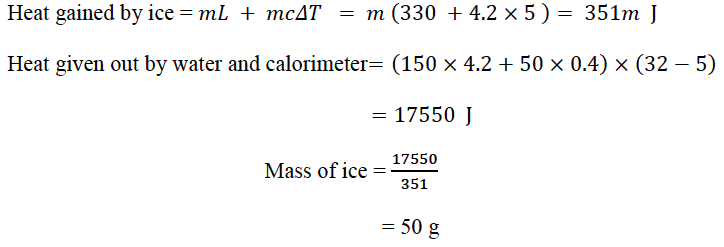(c) (i) Infra-red radiations of long wavelength

(iii) mass of the body

Specific heat capacity of the body

Change in temperature of the body (Any two correct factors)

### Question 7:

(a) A Lens forms an upright and diminished image of an object when the object is placed at the focal point of the given lens.

(i) Name the lens.

(ii) Draw a ray diagram to show the image formation.

(b) A ray of light travels from water to air as shown in the diagram given below:

####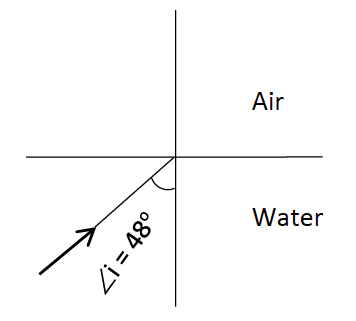(i) Copy the diagram and complete the path of the ray. Given the critical angle for

water is 48°.

(ii) State the condition so that total internal reflection occurs in the above diagram.

(c) The diagram below shows a point source P inside a water container. Four rays A, B, C, D starting from the source P are shown upto the water surface.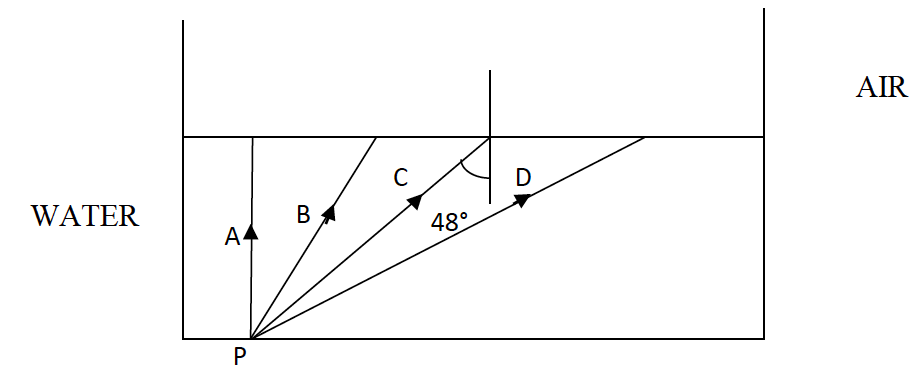(i) Show in the diagram the path of these rays after striking the water surface. The Critical Angle for water air surface is 48°.

(ii) Name the phenomenon which the rays B and D exhibit.

(a) (i) Concave lens

(ii) A ray shown parallel to the principal axis and appears to be coming from F2. A ray shown passing through optical centre and moving un-deviated.

Image shown correctly with object at the focus.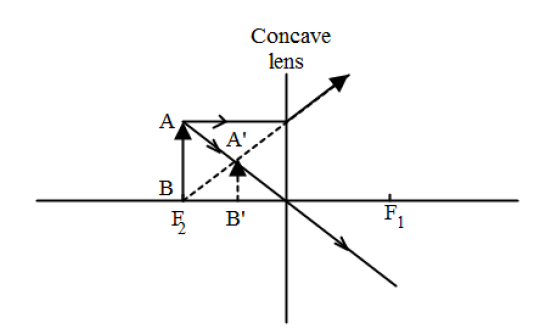(b) (i)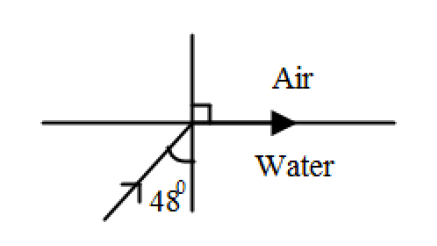(ii) ∠𝑖 should be greater than 48°.

(c) (i)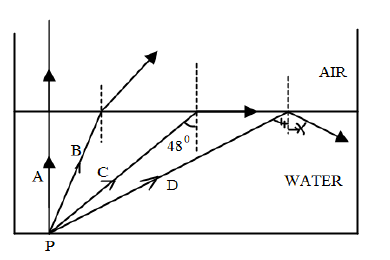(ii) Ray B undergoes refraction of light

Ray D undergoes total internal reflection of light.

### Question 8:

(a) Name the factor that determines:

(i) Loudness of the sound heard.

(ii) Quality of the note.

(iii) Pitch of the note.

(b) (i) What are damped vibrations?

(ii) Give one example of damped vibrations.

(iii) Name the phenomenon that causes a loud sound when the stem of a vibrating tuning fork is kept pressed on the surface of a table.

(c) (i) A wire of length 80 cm has a frequency of 256 Hz. Calculate the length of a similar wire under similar tension, which will have frequency 1024 Hz.

(ii) A certain sound has a frequency of 256 hertz and a wavelength of 1.3 m.

1. Calculate the speed with which this sound travels.
2. What difference would be felt by a listener between the above sound and another sound travelling at the same speed, but of wavelength 2.6 m?

(a) (i) Intensity/ amplitude of the sound wave

(ii) wave form/ number of overtones present in the waves

(iii) Frequency of the wave (Any other correct factor)

(b) (i) The periodic vibrations of decreasing amplitude due to the presence of a resistive force are called as damped vibrations.

(ii)

– A vibrating tuning fork in air

– Oscillations of a simple pendulum in air

– A slim branch of a tree pulled and released (Any one)

(iii) Forced vibration or resonance

(c)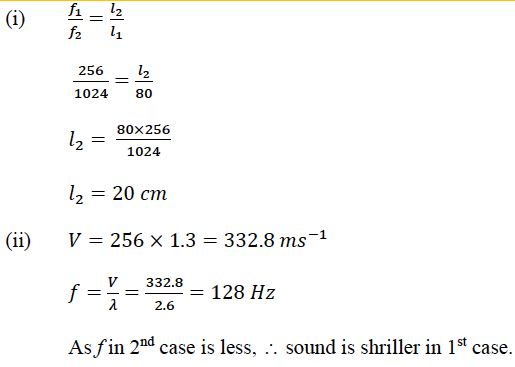### Question 9:

(a) (i) Name the colour code of the wire which is connected to the metallic body of an appliance.

(ii) Draw the diagram of a dual control switch when the appliance is switched ‘ON’.

(b) (i) Which particles are responsible for current in conductors?

(ii) To which wire of a cable in a power circuit should the metal case of a geyser be connected?

(iii) To which wire should the fuse be connected?

(c) (i) Explain the meaning of the statement ‘current rating of a fuse is 5A’.

(ii) In the transmission of power, the voltage of power generated at the generating stations is stepped up from 11kV to 132kV before it is transmitted. Why?

(a) (i) Green / Yellow

(ii)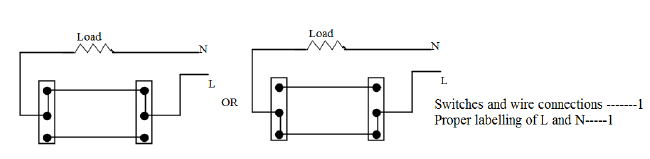(b) (i) Electrons

(ii) Earth wire

(iii) Live wire

(c) (i) It can afford a maximum current of 5A. If current in the circuit exceeds beyond 5A, fuse blows.

(ii) For given electric power, the current becomes low at a high voltage and therefore the loss of energy due to heating in the line wire becomes less.

### Question 10:

(a) Answer the following questions based on a hot cathode ray tube.

(i) Name the charged particles.

(ii) State the approximate voltage used to heat the filament.

(iii) What will happen to the beam when it passes through the electric field?

(b) State three factors on which the rate of emission of electrons from a metal surface depends.

(c) (i) What are free electrons?

(ii) Why do they not leave the metal surface on their own?

(iii) How can they be made to leave the metal surface? (State any two ways)

(a) (i) Cathode rays / high speed electrons

(ii) 6 V

(iii) The beam will get deflected towards the positive plate

(b) (i) Temperature of the metal surface

(ii) Surface area of the metal.

(iii) Nature of metal surface.

(c) (i) Electrons in outer orbits are attracted weakly by the nucleus and so they are loosely bound and are called free electrons.

(ii) They do not have sufficient kinetic energy.

(iii) By imparting heat energy or light energy or electrical energy to the metal surface.

We hope ICSE Class 10 Physics Question Paper Solution 2017 must have helped students in their exam preparation. We have also compiled the solutions of other subjects of ICSE Class 10 Previous Years Question Papers at one place. Students can access them by clicking here. Stay tuned to BYJU’S for the latest update on ICSE/CBSE/State Boards/Competitive exams and don’t forget to download the BYJU’S App.